# Foundations and Linear Equations in Precalculus: Help and Review Chapter Exam

Exam Instructions:

Choose your answers to the questions and click 'Next' to see the next set of questions. You can skip questions if you would like and come back to them later with the yellow "Go To First Skipped Question" button. When you have completed the practice exam, a green submit button will appear. Click it to see your results. Good luck!

### Page 2

#### Question 6 6. If the graph below has the equation Ax + By = -4, what must be true about A and B?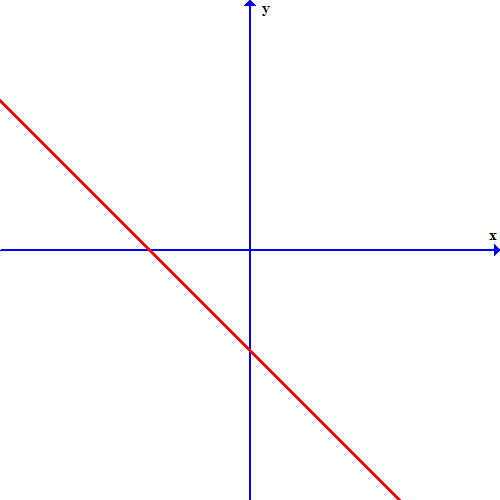#### Question 7 7. If the graph below has the equation Ax+By=C, which statement MUST be true?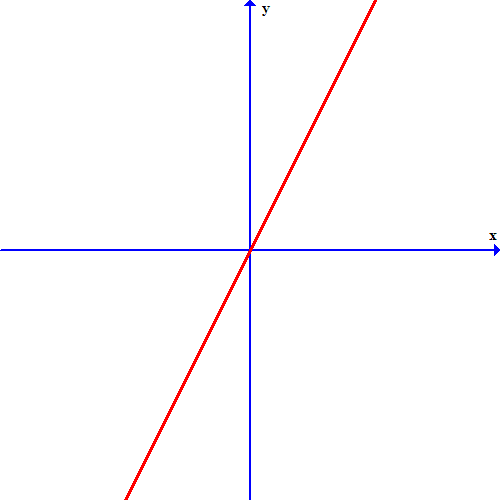#### Question 9 9. Which of these equations represents a line that is perpendicular to the line in the graph below?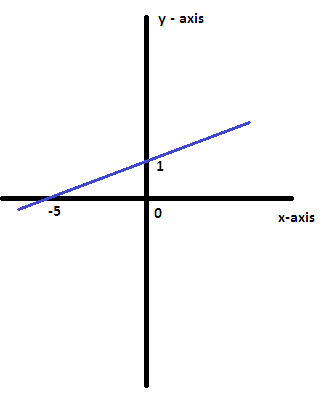### Page 3

#### Question 11 11. What is the slope-intercept form equation of the graph below?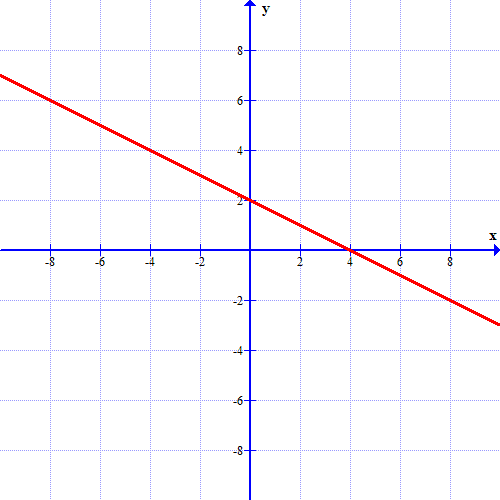### Page 4

#### Question 19 19. The equation for the following graph could be _____.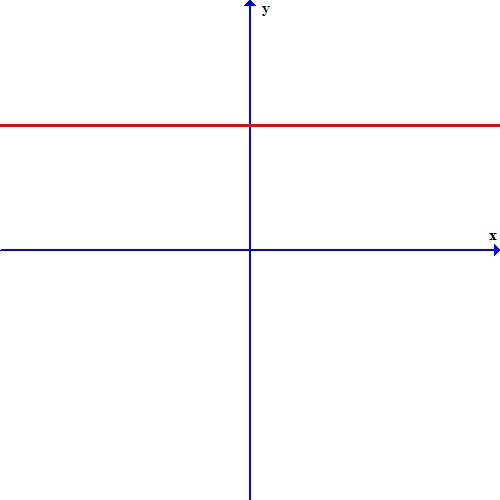### Page 6

#### Question 26 26. If the graph below was written in the slope intercept form, which of the following would be true?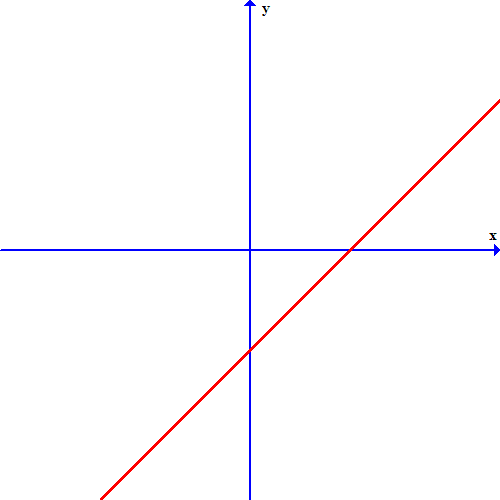#### Foundations and Linear Equations in Precalculus: Help and Review Chapter Exam Instructions

Choose your answers to the questions and click 'Next' to see the next set of questions. You can skip questions if you would like and come back to them later with the yellow "Go To First Skipped Question" button. When you have completed the practice exam, a green submit button will appear. Click it to see your results. Good luck!

Support